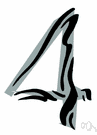quaternion

(redirected from Quarternion)
Also found in: Thesaurus, Encyclopedia.

qua·ter·ni·on

(kwə-tûr′nē-ən)
n.
1. A set of four persons or items.
2. Mathematics Any number of the form a + bi + cj + dk where a, b, c, and d are real numbers, ij = k, i2 = j2 = -1, and ij = -ji. Under addition and multiplication, quaternions have all the properties of a field, except multiplication is not commutative.

[Middle English quaternioun, from Late Latin quaterniō, quaterniōn-, from Latin quaternī, by fours, from quater, four times; see kwetwer- in Indo-European roots.]

quaternion

(kwəˈtɜːnɪən)
n
1. (Mathematics) maths a generalized complex number consisting of four components, x = x0 + x1i + x2j + x3k, where x, x0…x3 are real numbers and i2 = j2 = k2 = –1, ij = –ji = k, etc
2. another word for quaternary5
[C14: from Late Latin quaterniōn, from Latin quaternī four at a time]

qua•ter•ni•on

(kwəˈtɜr ni ən)

n.
1. a group or set of four persons or things.
2. a generalization of a complex number to four dimensions with three different imaginary units in which a number is represented as the sum of a real scalar and three real numbers multiplying each of the three imaginary units.
[1350–1400; Middle English quaternioun < Late Latin quaterniō= Latin quatern(ī) four at a time + -iō -ion]
ThesaurusAntonymsRelated WordsSynonymsLegend:
 Noun 1quaternion - the cardinal number that is the sum of three and onedigit, figure - one of the elements that collectively form a system of numeration; "0 and 1 are digits"
Translations
Quaternion
cuaternión
quaternion
quaternione
quaternion
kwaternion
References in periodicals archive ?
Arapostathis, "Quarternion feedback regulator for spacecraft eigenaxis rotations," Journal of Guidance, Control, and Dynamics, vol.
2a marks the start of an intact quarternion quire, indicating that the first folio had been cut from another set of pages and glued into this codex.
The nonlinear measurement model was obtained based on the principals of operation of the magnetometer and accelerometer and the properties of the quarternion vector space.

Site: Follow: Share:
Open / Close